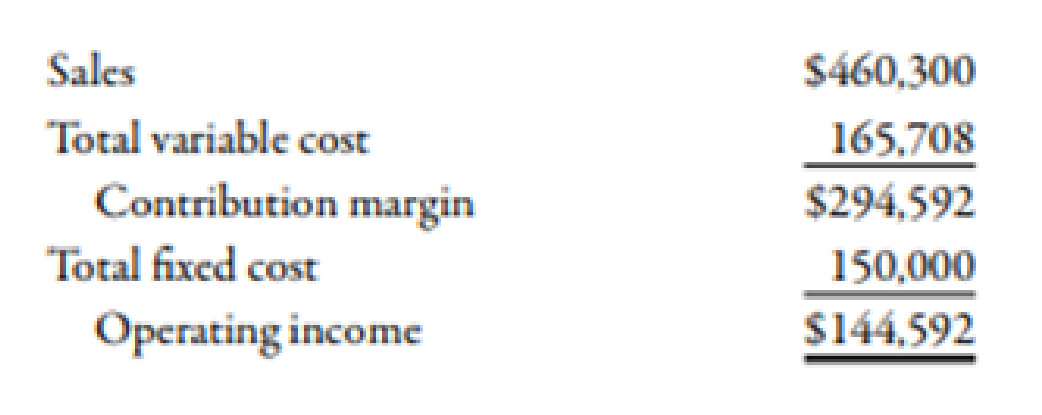Chapter 7, Problem 56P### Managerial Accounting: The Corners...

7th Edition
Maryanne M. Mowen + 2 others
ISBN: 9781337115773

#### Solutions

Chapter
Section### Managerial Accounting: The Corners...

7th Edition
Maryanne M. Mowen + 2 others
ISBN: 9781337115773
Textbook Problem
17 views

# Contribution Margin Ratio, Break-Even Sales, Operating LeverageElgart Company produces plastic mailboxes. The projected income statement for the coming year follows:Required: 1. Compute the contribution margin ratio for the mailboxes. 2. How much revenue must Elgart earn in order to break even? 3. What is the effect on the contribution margin ratio if the unit selling price and unit variable cost each increase by 15%? 4. CONCEPTUAL CONNECTION Suppose that management has decided to give a 4% commission on all sales. The projected income statement does not reflect this commission. Recompute the contribution margin ratio, assuming that the commission will be paid. What effect does this have on the break-even point? 5. CONCEPTUAL CONNECTION If the commission is paid as described in Requirement 4, management expects sales revenues to increase by $80,000. How will this affect operating leverage? Is it a sound decision to implement the commission? Support your answer with appropriate computations. 1. To determine Calculate the contribution margin ratio. Explanation Contribution Margin Ratio: The sales percentage remaining after covering the amount of total variable cost is known as the contribution margin ratio. It is the available sales dollar percentage which will be used to cover the total fixed cost. Use the following formula to calculate contribution margin ratio: Contribution margin ratio=Contribution marginSales Substitute$294,592 for contribution margin and \$460,300 for sales in the above formula

2.

To determine

Calculate the revenue that Company E will earn at break-even.

3.

To determine

Describe the effect on contribution margin if the selling price per unit and variable cost per unit are increased by 15%.

4.

To determine

Calculate the contribution margin ratio after considering a commission. Also, describe its effect on the break-even point.

5.

To determine

Calculate the effect of the increase in sales after paying commission, on operating leverage.

### Still sussing out bartleby?

Check out a sample textbook solution.

See a sample solution

#### The Solution to Your Study Problems

Bartleby provides explanations to thousands of textbook problems written by our experts, many with advanced degrees!

Get Started